# Thermal Expansion of a BeamThis test lets you check analysis results for the thermal expansion of a beam, in the context of a static case. You will use 3D mesh with tetrahedron and hexahedron elements.Reference:

Thermal Expansion Theory

##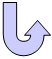Specifications

### Geometry Specifications

 Length: L = 1000 mm Model 3D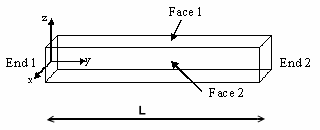Model 2D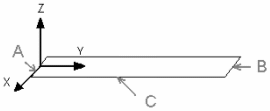Model 1D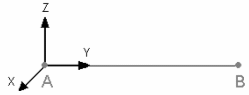Section (square): a = 100 mm

### Analysis Specifications

 Young Modulus (material): E = 200 GPa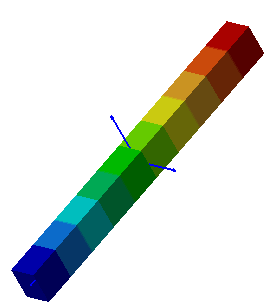Model 3D - Case 1 Poisson's Ratio (material): ν = 0.266 Thermal Expansion Coefficient: α= 11.7 x 10-6/° Mesh Specifications: Size = 50 mm Restraints: 3D Model Case 1: Ty = 0 on End 1, Tz = 0 on Face 1 and Tx = 0 on Face 2 Case 2: Ty  = 0 on End 1 and End 2, Tz = 0 on Face 1 and Tx = 0 on Face 2 Case 3: Ty  = 0 on End 1, Tz = 0 on Face 1 and Tx = 0 on Face 2 2D Model Case 1: Ty = 0 on Edge A, Tx = 0 on Edge C and Tz = 0 on the surface Case 2: Ty  = 0 on Edge A and Edge B, Tx = 0 on Edge C and Tz = 0 on the surface Case 3: Ty  = 0 on Edge A, Tx = 0 on Edge C and Tz = 0 on the surface 1D Model Case 1: Tz = 0 on A Case 2: Ty  = 0 on A and B Case 3: Ty  = 0 on A Loads: Case 1: Temperature Field T1 = 325 K Case 2: Temperature Field T1 = 325 K Case 3: Temperature Field T1 = 325 K and a Surface force density Fy = -5.85e+007 N_m2 The environment temperature is T0 = 300 K

##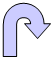Results

• The analytic solution is:
 Case 1: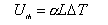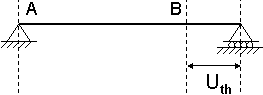Case 2: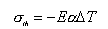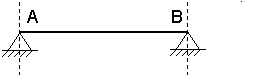Case 3: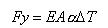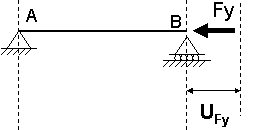• The computed results correspond to:
• Uth: Maximal elongation at End 2 under thermal expansion effect.
• σ22: Mean stress tensor of the beam, when the two extremities are clamped.
• Uth- UFy: Maximal final displacement at End 2, when the force Fy is opposed to the thermal expansion.

The computed results are the same for the following elements: TE4, TE10, HE8, HE20, TR3, TR6, QD4, QD8, bar (linear and parabolic):

 Case Quantity Analytical solution Computed results Case 1 Uth[mm] 0.2925 0.2925 Case 2 σ22 [MPa] -58.5 -58.5 Case 3 Uth- UFy [mm] 0 0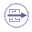To Perform the Test:

The Thermal_expansion_of_a_beamTE10.CATAnalysis document presents the complete analysis of this case, computed with a parabolic tetrahedron elements (TE10).

The Thermal_expansion_of_a_beamHE20.CATAnalysis document presents the complete analysis of this case, computed with a mesh formed of parabolic hexahedron elements (HE20).

To compute the case with linear elements, proceed as follow:

1. Open one of the CATAnalysis documents.

2. Enter the Advanced Meshing Tools workbench.

3. In the specification tree, double-click the mesh:

 Volume mesh  for linear tetrahedron elements (TE4). Surface mesh for linear hexahedron elements (HE8). The Global Parameters dialog box appears.
4. Select the Linear element type.

5. Compute the case in the Generative Structural Analysis workbench.